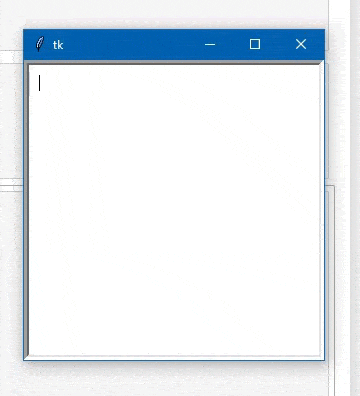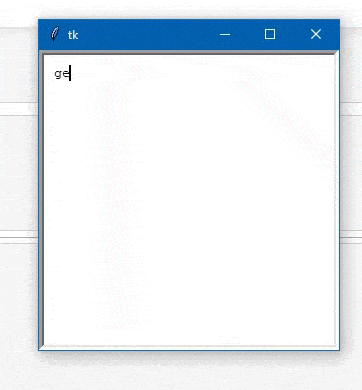# How to wrap text within Tkinter Text Box?

• Last Updated : 26 Mar, 2021

In this article, we will see that how can we wrap the text in the TKinter Text-Box using the Tkinter module Called textWrap Module. The textwrap module can be used for wrapping and formatting plain text. This module provides formatting of the text by adjusting the line breaks in the input paragraph.

Example 1:

Firstly We will import the Tkinter Library to the Code then we will declare it as a root for our Window then after declaring the size of the window we will call the function in the Tkinter called text() which will provide the Text box in that Window then declare the size of the Text Box then pack to the window or combined the textBox to the Window.

Below is the implementation:

## Python3

 `# import tkinter module ``from` `tkinter ``import` `*`       `  ` `# Create Object``root ``=` `Tk() ``  ` `# Initialize tkinter window with dimensions 100x100             ``root.geometry(``'300x300'``)     ``  ` `text``=``Text(root,``          ``width ``=` `50``,``          ``height ``=` `50``,``          ``padx ``=` `10``, ``          ``pady ``=` `10``)`` ` `# pack the text-Aera in the window``text.pack()`` ` `root.mainloop()`

Output:Example 2:

Here We have the Problem like at the end of the box when we write something then it breaks the text to the new line. Generally to wrap the text we use the Text(wrap=word). Here is the basic idea about how we use the word=wrap

Use the wrap=WORD option. Here’s an example:

```from tkinter import *
root = Tk()
t = Text(wrap=WORD)
t.pack()
root.mainloop()```

Below is the implementation:

## Python3

 `# import tkinter module ``from` `tkinter ``import` `*`       `  ` `# Create Object``root ``=` `Tk() ``  ` `# Initialize tkinter window with dimensions 100x100             ``root.geometry(``'300x300'``)     ``  ` `text``=``Text(root, width ``=` `50``, height ``=` `50``, ``          ``wrap ``=` `WORD, padx ``=` `10``, pady ``=` `10``)`` ` `# pack the text-Aera in the window``text.pack()`` ` `root.mainloop()`My Personal Notes arrow_drop_up